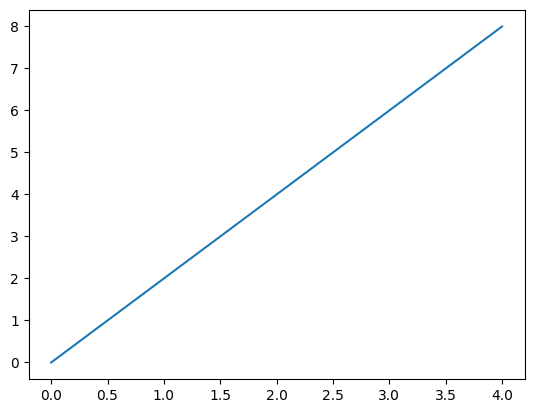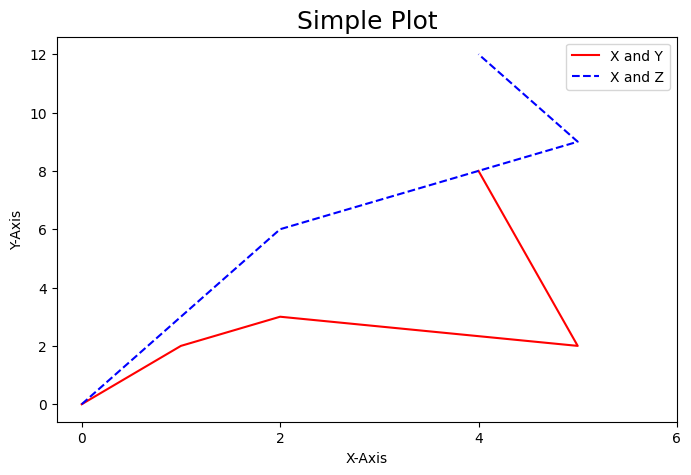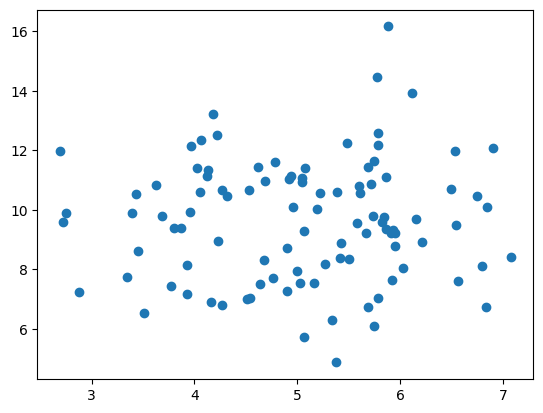# Introduction to Python: Numpy, Pandas, Matplotlib¶

Authors:

Tahmidul Azom Sany (tsany@gmu.edu)

NumPy, pandas, and Matplotlib are popular Python libraries used in climate science for data analysis, manipulation, and visualization. NumPy provides efficient arrays for handling large datasets and mathematical operations. Pandas offers data structures for tabular data manipulation, while Matplotlib allows for creating various types of visualizations. Together, these libraries provide a comprehensive toolkit for climate scientists to handle data, perform analysis, and create visualizations for interpreting and communicating climate-related findings. In this tutorial we will explore some of the features of these three packages in Python.

Prerequisite: It is recommended for users to have a basic understanding of the Python programming language before proceeding with this tutorial.

## 1. Numpy ¶

In :
# Importing NumPy library
import numpy as np

In :
# Creating a numpy array
arr = np.array([1, 2, 3, 4, 5])
arr # or print(arr) both will print the array created

Out:
array([1, 2, 3, 4, 5])
In :
# Accessing elements of a numpy array
print("Element at index 0:", arr)
print("Element at index 2:", arr)
print("Element at last index :", arr[-1])

Element at index 0: 1
Element at index 2: 3
Element at last index : 5

In :
# Slicing
print("All Element in that array:", arr[:])
print("First three Element :", arr[:3])
print("Last three Element :", arr[2:])

All Element in that array: [1 2 3 4 5]
First three Element : [1 2 3]
Last three Element : [3 4 5]

In :
# Performing basic operations on the array
print("Sum of elements in the array:", np.sum(arr))
print("Product of elements in the array:", np.prod(arr))
print("Mean of elements in the array:", np.mean(arr))
print("Minimum value in the array:", np.min(arr))
print("Maximum value in the array:", np.max(arr))
print("Square root of elements in the array:", np.sqrt(arr))
print("Standard deviation of elements in the array:", np.std(arr))
print("Array sorted in ascending order:", np.sort(arr))

Sum of elements in the array: 15
Product of elements in the array: 120
Mean of elements in the array: 3.0
Minimum value in the array: 1
Maximum value in the array: 5
Square root of elements in the array: [1.         1.41421356 1.73205081 2.         2.23606798]
Standard deviation of elements in the array: 1.4142135623730951
Array sorted in ascending order: [1 2 3 4 5]

In :
# Creating a 2D numpy array
matrix = np.array([[1, 2, 3], [4, 5, 6], [7, 8, 9]])
print("Shape of the array: ", matrix.shape)
matrix

Shape of the array:  (3, 3)

Out:
array([[1, 2, 3],
[4, 5, 6],
[7, 8, 9]])
In :
# Accessing elements of a 2D numpy array
print("Element at row 0 and column 1:", matrix)
print("Element at row 2 and column 2:", matrix)

Element at row 0 and column 1: 2
Element at row 2 and column 2: 9

In :
# Performing operations on 2D numpy arrays (note the axis value)
print("Sum of elements in each row:", np.sum(matrix, axis=1))
print("Sum of elements in each column:", np.sum(matrix, axis=0))

Sum of elements in each row: [ 6 15 24]
Sum of elements in each column: [12 15 18]

In :
# Performing operations on the matrix
print("Sum of elements in the matrix:", np.sum(matrix))
print("Product of elements in the matrix:", np.prod(matrix))
print("Mean of elements in the matrix:", np.mean(matrix))
print("Minimum value in the matrix:", np.min(matrix))
print("Maximum value in the matrix:", np.max(matrix))
print("Transpose of the matrix:\n", np.transpose(matrix)) # "\n" is used for line space
print("Matrix sorted in ascending order along rows:\n", np.sort(matrix, axis=1))
print("Matrix sorted in ascending order along columns:\n", np.sort(matrix, axis=0))

Sum of elements in the matrix: 45
Product of elements in the matrix: 362880
Mean of elements in the matrix: 5.0
Minimum value in the matrix: 1
Maximum value in the matrix: 9
Transpose of the matrix:
[[1 4 7]
[2 5 8]
[3 6 9]]
Matrix sorted in ascending order along rows:
[[1 2 3]
[4 5 6]
[7 8 9]]
Matrix sorted in ascending order along columns:
[[1 2 3]
[4 5 6]
[7 8 9]]

In :
# Reshaping the array (one of the mostly used features)
array = np.arange(1, 11) # create a array
print("inital array: ", array)

array_reshaped = np.reshape(array, (2, 5))
print("final array after reshaping: \n ", array_reshaped)

inital array:  [ 1  2  3  4  5  6  7  8  9 10]
final array after reshaping:
[[ 1  2  3  4  5]
[ 6  7  8  9 10]]

In :
# Performing mathematical operations on numpy arrays
arr2 = np.array([1, 3, 5, 7, 9])
arr3 = np.array([2, 4, 6, 8, 10])
print("Subtraction of arrays:", np.subtract(arr2, arr3))
print("Multiplication of arrays:", np.multiply(arr2, arr3))
print("Division of arrays:", np.divide(arr2, arr3))

Addition of arrays: [ 3  7 11 15 19]
Subtraction of arrays: [-1 -1 -1 -1 -1]
Multiplication of arrays: [ 2 12 30 56 90]
Division of arrays: [0.5        0.75       0.83333333 0.875      0.9       ]

In :
# Using numpy functions to perform mathematical operations
print("Square root of elements:", np.sqrt(arr2))
print("Exponential of elements:", np.exp(arr2))
print("Logarithm of elements:", np.log(arr2))

Square root of elements: [1.         1.73205081 2.23606798 2.64575131 3.        ]
Exponential of elements: [2.71828183e+00 2.00855369e+01 1.48413159e+02 1.09663316e+03
8.10308393e+03]
Logarithm of elements: [0.         1.09861229 1.60943791 1.94591015 2.19722458]


## 2. Pandas ¶

In :
# Importing the Pandas library
import pandas as pd

In :
# Creating a sample DataFrame
name =  ['Alice', 'Bob', 'Charlie', 'Dave', 'Eve']
age = [25, 30, 35, 40, 45]
city = ['New York', 'Los Angeles', 'Chicago', 'San Francisco', 'Seattle']

df = pd.DataFrame() # Create a empty dataframe
df['Name'] = name # Adding values to Dataframe, you can name the column anything
df['Age'] = age
df['City'] = city

# Displaying the DataFrame
print("Original DataFrame:")
df

Original DataFrame:

Out:
Name Age City
0 Alice 25 New York
1 Bob 30 Los Angeles
2 Charlie 35 Chicago
3 Dave 40 San Francisco
4 Eve 45 Seattle
In :
# Accessing columns
print("\nAccessing Columns:")
print(df['Name'])        # Accessing a single column
print(df[['Name', 'Age']])   # Accessing multiple columns

Accessing Columns:
0      Alice
1        Bob
2    Charlie
3       Dave
4        Eve
Name: Name, dtype: object
Name  Age
0    Alice   25
1      Bob   30
2  Charlie   35
3     Dave   40
4      Eve   45

In :
# Accessing rows (Try run one by one for better understanding)
print("\nAccessing Rows:")
print(df.loc)         # Accessing a single row by label
print(df.iloc)        # Accessing a single row by index
print(df[1:4])           # Accessing multiple rows using slicing

Accessing Rows:
Name    Charlie
Age          35
City    Chicago
Name: 2, dtype: object
Name    Charlie
Age          35
City    Chicago
Name: 2, dtype: object
Name  Age           City
1      Bob   30    Los Angeles
2  Charlie   35        Chicago
3     Dave   40  San Francisco

In :
# Data manipulation
print("\nData Manipulation:")
df['City'] = df['City'].str.upper()   # Modifying values in a column
df['Country'] = 'USA'                 # Adding a new column with constant value
df.drop('Age', axis=1, inplace=True)  # Removing a column
df.rename(columns={'Name': 'Full Name'}, inplace=True)  # Renaming a column
df.sort_values(by='Full Name', inplace=True)  # Sorting DataFrame by a column
df.reset_index(drop=True, inplace=True)      # Resetting index after sorting
df['City'] = df['City'].apply(lambda x: x.replace(' ', '-'))  # Applying a function to a column
df

Data Manipulation:

Out:
Full Name City Country
0 Alice NEW-YORK USA
1 Bob LOS-ANGELES USA
2 Charlie CHICAGO USA
3 Dave SAN-FRANCISCO USA
4 Eve SEATTLE USA
In :
# Data analysis
print("\nData Analysis:")
print(df.describe())          # Descriptive statistics of numeric columns
print("----------------------------------------")
print(df['Full Name'].value_counts())   # Counting occurrences of values in a column

Data Analysis:
Full Name      City Country
count          5         5       5
unique         5         5       1
top        Alice  NEW-YORK     USA
freq           1         1       5
----------------------------------------
Alice      1
Bob        1
Charlie    1
Dave       1
Eve        1
Name: Full Name, dtype: int64


## 3. Matplotlib ¶

In :
import matplotlib.pyplot as plt

In :
#Prepare the data
x = [0,1,2,3,4]
y = [0,2,4,6,8]

#ploting data
plt.plot(x,y)

#show graph
plt.show()In :
x = [0,1,2,5,4]
y = [0,2,3,2,8]
z = [0,3,6,9,12]

#figure size
plt.figure(figsize=(8,5), dpi= 100) #dot per inch or pixel per inch

#ploting data
plt.plot(x,y, color ='r', label = 'X and Y')
plt.plot(x,z, 'b--', label = 'X and Z')

#labeling x,y axis
plt.xlabel("X-Axis")
plt.ylabel("Y-Axis")

#tickmark
plt.xticks([0,2,4,6])

#Provide title
plt.title("Simple Plot", fontsize = 18)

plt.legend()
plt.show()In :
# Plotting Scatterplot
x = np.random.normal(5.0, 1.0, 100)
y = np.random.normal(10.0, 2.0, 100)

plt.scatter(x, y)
plt.show()